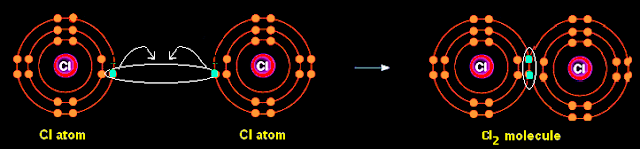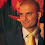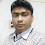Lewis Structures|Octet Rule: A Simple Method to write Lewis Structures | Chemistry Net

# Lewis Structures|Octet Rule: A Simple Method to write Lewis Structures

### A Simple and General Method to write Lewis Structures

Lewis structures constitute an overwhelming majority of the structures that students encounter in courses in general chemistry, organic chemistry, or biochemistry. Hence, the writing of Lewis structures corresponding to a given molecular formula is an important exercise for them. The ability of students to write these structures correctly and then to calculate the formal charges of the atoms involved, is a prerequisite to understanding such topics as:

·         Reπactivity
·         Isomerism and molecular geometry.

Since many chemistry textbooks do not give easy procedures for writing such structures we present a set of instructions for writing Lewis structures (1-3).

But at first let’s see the following:

#### Which are the essential propositions of the Lewis theory for bonding?

Chemical bonding is at the heart of understanding chemistry. In 1916, G.N. Lewis published a paper on the chemical bond in nonionic substances, entitled “The Atom and the Molecule” ( ). The Bohr theory of the atom, proposed a few years earlier, suggested the possibility of a rational explanation for the patterns of chemical combination shown by the elements that had earlier led to the periodic table. G.N. Lewis using essentially empirical concepts along with his chemical intuition combined observations at the time about chemical bonding. The theory he proposed not only was essential in understanding how elements bonded but also provided a visual representation for them the so called Lewis symbols and the Lewis dot structures.

Lewis symbols: A Lewis symbol consists of the element symbol surrounded by dots to represent the number of electrons in the outer shell. The number of electrons in the outer shell is correlated by simply reading the group number.

The Lewis symbol for fluorine is shown below:Figure 1: Lewis symbol for the atom of fluorine. The valence electrons are shown as dots around the chemical symbol of fluorine

Lewis structures (or electron dot structures) are a simplistic way of representing the electrons in molecules. Valence electrons – electrons of the outermost orbital of the atom -  are represented as dots surrounding the symbol of an element
i.e. the Lewis structure for the ion of CN-

The essential propositions of the Lewis theory for bonding were based on the fact that atoms with 8 electrons in their outer shell (like the noble gases) exhibit increased stability ( octet rule ).

·    Chemical bonds are formed when atoms transfer valence electrons to attain noble gas electron configutations ( octet of electrons in the outermost orbital, duet of electrons in the case of hydrogen). The type of bond formed in this case is called an ionic bond.
·   Chemical bonds are formed when atoms share valence electrons to attain noble gas electron configurations . The type of bond formed in this case is called a covalent bond.

#### Which are the types of bonds proposed by Lewis?

There are two basic types of bonding proposed in the Lewis theory:

·        Ionic bonding: This type of bonding occurs when outer electron(s) are transferred from one atom to an other so that both of them attain an octet of electrons in their outermost shell. The two atoms are held together by electrostatic forces

Ionic bonding occurs between metals and non-metals. The metal gives its outer electrons (it gains a positive charge since it looses electrons) to the non-metal (it gains a negative charge). As the ions formed have opposite charges they will attract each other and this attractive or electrostatic force is called an ionic bond.

﻿﻿Figure 2: Schematic representation of the formation of an ionic bond by the transfer of an electron from the outer shell of the Na atom to the outer shell of the Cl.

Please see the following video on the formation of ionic bonds:

·       Covalent bonding: This type of bonding forms when two non-metal atoms share a pair of outer electrons so that both of them attain an octet of electrons in their outermost shell.Figure 3: Schematic representation of the formation of a covalent bond between two chlorine atoms. Each atom contributes an outer electron for the formation of a shared pair of electrons ( covalent bond ). Both atoms become more stable since they achieve an octet of electrons in their outer shell.
Covalent bonding occurs between non-metals. Each atom contributes one electron for each covalent bond formed.

#### Is there a simple procedure enabling one to write down correctly and rapidly Lewis structures for compounds formed by elements of the three first rows of the periodic table?

Compounds formed by elements of the first three rows of the periodic table generally obey the Octet Rule . However, until expertise has been gained, confusion often arises concerning:

·         The correct number of multiple bonds to use
·         The correct number of valence electrons to use
·         The placing of formal charges
Therefore, it is not as straightforward as it seems  when it comes to write down a Lewis structure

A simple procedure for writing Lewis structures for molecules that eliminates all the above problems is as follows (3):

1)      Find the central atom of the molecule. Connect the central atom with the other atoms of the molecule with single bonds.

The central atom usually has a “subscript” of 1 in the molecular formula. If there are present two atoms with “subscript” 1 then the central atom is the less electronegative of the two (for an electronegativity table see here) .
The central atom can not be a hydrogen atom

i.e. Consider the molecule with a general molecular formula ABx. Identify the central atom.
The central atom is the A atom since it has a subscript equal to 1.

2)      Calculate the number of electrons which must be shared through π bonds (pi bonding), P in the molecule:

P = 6n + 2 – V                            (1)

Where n  is the number of atoms in the molecule excluding the hydrogen atoms

V is the total number of valence electrons in the molecule  =
= ( sum of group numbers of  the atoms in the molecule) – charge

3)      Add double or triple bonds to the structure in Step 1 according to the results obtained in Step 2. Add unshared pairs of electrons around each atom so that all of them have octets around them (except for the H atom)

4)      Calculate the formal charges of the atoms in the molecule as follows: i) Any atom having its normal valency number of bonds will be electrically neutral; any atom which does not have its normal valency number of bonds will have a formal charge  ii) The formal charge may be calculated by subtracting the number of electrons “owned” by the element from its group number

Formal Charge of Element = Group Number Total number of electrons “owned” by the atom concerned                                                                                             (2)

As an example let us write the Lewis structure for the CN- ion:

Step1: Since the molecule has 2 atoms and it is linear there is no central atom. Connect the 2 atoms with a single bond.

Step 2: Calculate the # of electrons in p bonds (multiple bonds) using formula (1):

Where n in this case is 2 since CN- consists of two atoms
Where V = (4 + 5) – (-1) = 10
Therefore, P = 6 * 2 + 2 – 10 = 4      So there are 4 π (pi) electrons in CN-   and that means either 2 double  or 1 triple bond.

Since there are only 2 atoms there is no possibility for  2 double bonds
The 2 atoms are joined together with a triple bond. Therefore a draw for the Lewis structure of CN- is as follows:Figure 4: Lewis structures for the CN ion. Structure 1 is the most plausible since there is an octet of electrons around each element and there is less formal charge compared to structure 3.  Structure 2 is highly unfavorable since there is no octet of electrons around the C atom

﻿
Additional examples on this subject are given in the article “Simple Procedure for writing Lewis Structures – Examples”.

Relevant Posts - Relevant Videos

References

1. G.N. Lewis, J.A.C.S, 38, 762-785, (1916)
2.  E. C. McGoran, J. Chem. Educ., 68, 19-23 (1991)
3. A.B.P. Lever, J. Chem. Educ., 49, 819-821, (1972)

Key Terms

Lewis structures, how can I write the Lewis structure, pi and, for the draw, Lewis symbols, electron dot structures, ionic bonding, covalent bonding, structure de lewis, representation de Lewisestructura de Lewis, 路易士结构, formule de Lewis

1.How can I use the above method in order to draw the Lewis structure of SO3?

1.The above method was used to draw the Lewis electron dot structure of SO3. Please see the following post: "Lewis structures of SO3" http://chem-net.blogspot.com/2011/05/simple-procedure-for-writing-lewis_27.html

2.Can the above method be used to draw the Lewis structure of cyclic molecules?

3.Clearly cyclic molecules can also be handled by this procedure by taking into account the fact that an n atom cyclic molecule will share 2n (rather 2(n-1)) σ electrons

4.Can I use the above method to draw Lewis structures in which more than eight electrons are around an atom?

1.Lewis structures in which more than 8 electrons surround an atom are possible if this atom is that of an element found in the third row or a subsequent row of the periodic table. The simple method given above can be used to write Lewis structures with expanded octets. Please see the case of the sulfate ion:
http://chem-net.blogspot.com/2013/02/electron-dot-structure-of-sulfate-ion.html

5.Many thanks for this excellent guide to drawing Lewis structures. Just one question, how did you arrive at the formula for calculating the number of pi electrons?

1.6.Thanks. I feel confident this article will be very useful in my up-coming exam.

Regards.

7.Pls can u explain benzene 's structure using this

8.In PCL5 .. P (Pi Electrons) turns out to be -2 (6*6+2 - 5*7+5). What does this mean? How should one proceed in such situation?

9.SOCl2 how can we draw it ?
the total valence is 26 and the P=6n+2-v is also 26 which gives ZERO ..!!

1.I have it as an example of an expanded octet. Please check at: http://chem-net.blogspot.com/2013/06/lewis-dot-structure-thionyl-chloride.html

10.did not understand at all.... pi bond formula

11.Why the negative charge is on carbon atom?why Will it be wrong if we put it on the nitrogen atom? Please explain.

12.I really enjoyed reading your article. I found this as an informative and interesting post, so i think it is very useful and knowledgeable. I would like to thank you for the effort you have made in writing this article.